# IBPS PO Quantitative Aptitude Quiz- 25

## IBPS PO Quantitative Aptitude Quiz- 25

Quantitative Aptitude is the most significant part of almost all competitive exams. Many candidates find difficulty in solving Quantitative Aptitude Questions. The only way to ace this section is to practice as many questions as possible. And, to help you practice more and more questions, we have come up with the IBPS PO Pre Quantitative Aptitude Quiz. This IBPS PO Pre Quantitative Aptitude Quiz is completely Free. This IBPS PO Pre Quantitative Aptitude Quiz includes a variety of questions that are usually asked in preliminary and main exams of various banking exams. Candidates will be provided with a detailed solution for each question in this IBPS PO Pre Quantitative Aptitude Quiz. This IBPS PO Pre Quantitative Aptitude Quiz will assist candidates in better preparing for upcoming exams.

Directions (1-5): The following bar graph shows the production of cars in 6 months by 2 companies (A and B). Read the graph carefully and answer the following questions.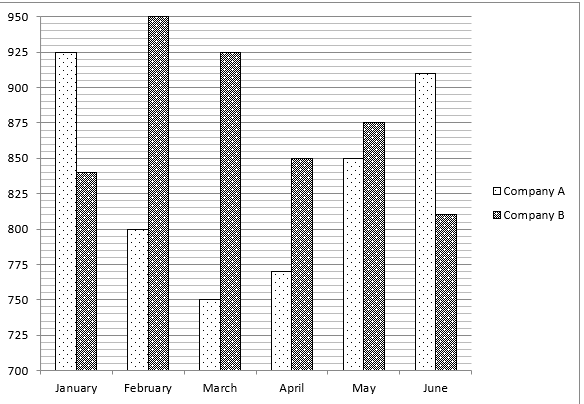1. If in July there is an increase of 800/91 % in the production of car of company A with respect to that of in June and there is a decrease of 1100/81 % in the production of car of company B with respect to that of in June then find the difference between the production of car of company A in July to that of company B in July.
(a) 290
(b) 300
(c) 275
(d) 270
(e) None of these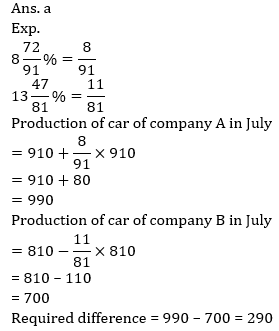2. Find the ratio between the total production of cars of company A from Feb to Apr and the total production of cars of company B from Apr to June.
(a) 507 : 464
(b) 464 : 507
(c) 275 : 119
(d) 507 : 23
(e) None of these3. The total production of cars of company B in Jan and June together are what percentage more/less than the total production of cars of company A in Apr and May together?4. Out of the total production of cars of company A from Jan to June together, 2450 cars are defective then the defective cars of company A from Jan to June together is what percent of the total production of cars of company B from Jan to June together?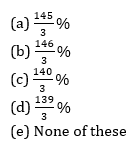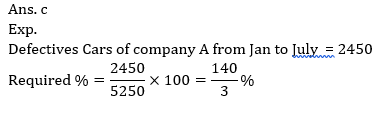5. Find the ratio between the average of total production of cars of company A and company B in Mar to the average of cars of company A and company B in June.
(a) 507 : 464
(b) 464 : 507
(c) 275 : 119
(d) 507 : 239
(e) 335 : 344Directions (6-10): In the following questions two equations numbered (I) and (II) are given. You have to solve both equations and Give answer

6. I. x² – 25x + 156 = 0
II. y² – 21y + 108 = 0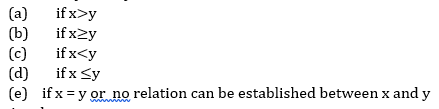Ans. b
Exp.
I. x² – 25x + 156 = 0
x² – 13x – 12x + 156 = 0
x = 13, 12
II. y² – 21y + 108 = 0
y² – 12y – 9y + 108 = 0
y = 12, 9
x ≥ y

7. I. 2x² – 7x – 15 = 0
II. 2y² +15y + 25 = 0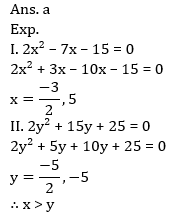8. I. 20x² + 27x + 9 = 0
II. 6y² + 29y + 35 = 0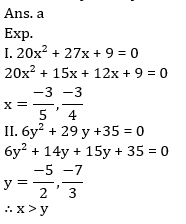9. I. 8x + 7y = 42
II. 5x+ 3y = 1810. I. 3x² + 25x – 18 = 0

II. 2y² + 15y + 27 = 0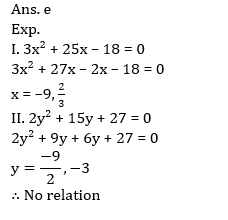###Click to Buy Bank MahaCombo Package

Recommended PDF’s for:

#### Most important PDF’s for Bank, SSC, Railway and Other Government Exam : Download PDF Now

AATMA-NIRBHAR Series- Static GK/Awareness Practice Ebook PDF Get PDF here
The Banking Awareness 500 MCQs E-book| Bilingual (Hindi + English) Get PDF here
AATMA-NIRBHAR Series- Banking Awareness Practice Ebook PDF Get PDF here
Computer Awareness Capsule 2.O Get PDF here
AATMA-NIRBHAR Series Quantitative Aptitude Topic-Wise PDF Get PDF here
AATMA-NIRBHAR Series Reasoning Topic-Wise PDF Get PDF Here
Memory Based Puzzle E-book | 2016-19 Exams Covered Get PDF here
Caselet Data Interpretation 200 Questions Get PDF here
Puzzle & Seating Arrangement E-Book for BANK PO MAINS (Vol-1) Get PDF here
ARITHMETIC DATA INTERPRETATION 2.O E-book Get PDF here

3